Thermodynamics and Propulsion

5.5 Calculation of Entropy Change in Some Basic Processes

1. Heat transfer from, or to, a heat reservoir.

A heat reservoir (Figure 5.3) is a constant temperature heat source or sink. Because the temperature is uniform, there is no heat transfer across a finite temperature difference and the heat exchange is reversible. From the definition of entropy (),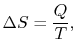where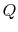is the heat into the reservoir (defined here as positive if heat flows into the reservoir.)2. Heat transfer between two heat reservoirs

The entropy change of the two reservoirs in Figure 5.4 is the sum of the entropy change of each. If the high temperature reservoir is at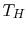and the low temperature reservoir is at, the total entropy change is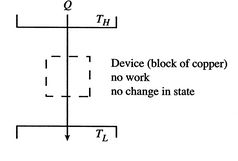The second law says that the entropy change must be equal to or greater than zero. This corresponds to the statement that heat must flow from the higher temperature source to the lower temperature source. This is one of the statements of the second law given in Section 5.1.

Muddy Points

In the single reservoir example, why can the entropy decrease? (MP 5.6)

Why does the entropy of a heat reservoir change if the temperature stays the same? (MP 5.7)

How can the heat transfer from or to a heat reservoir be reversible? (MP 5.8)

How canbe less than zero in any process? Doesn't entropy always increase? (MP 5.9)

If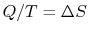for a reservoir, could you addto any size reservoir and still get the same? (MP 5.10)

3. Possibility of obtaining work from a single heat reservoir

We can regard the process proposed in Figure 5.5 as the absorption of heat,, by a device or system, operating in a cycle, rejecting no heat, and producing work. The total entropy change is the sum of the change in the reservoir, the system or device, and the surroundings. The entropy change of the reservoir is. The entropy change of the device is zero, because we are considering a complete cycle (return to initial state) and entropy is a function of state. The surroundings receive work only so the entropy change of the surroundings is zero. The total entropy change is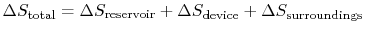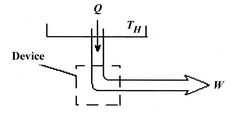The total entropy change in the proposed process is thus less than zero,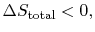which is not possible. The second law thus tells us that we cannot get work from a single reservoir only. The only'' is important; it means without any other changes occurring. This is the other statement of the second law we saw in Section 5.1.

Muddy Points

What is the difference between the isothermal expansion of a piston and the (forbidden) production of work using a single reservoir? (MP 5.11)

For the work from a single heat reservoir'' example, how do we know there is no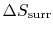? (MP 5.12)

How does a cycle produce zero? I thought that the whole thing about cycles was an entropy that the designers try to minimize. (MP 5.13)

4. Entropy changes in the hot brick problem''

 [Temperature equalization of two bricks]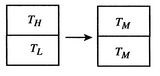[Reservoirs used in reversible state transformations]We can examine in a more quantitative manner the changes that occurred when we put the two bricks together, as depicted in Figure 5.6(a). The process by which the two bricks come to the same temperature is not a reversible one, so we need to devise a reversible path. To do this imagine a large number of heat reservoirs at varying temperatures spanning the range, as in Figure 5.6(b). The bricks are put in contact with them sequentially to raise the temperature of one and lower the temperature of the other in a reversible manner. The heat exchange at any of these steps is. For the high temperature brick, the entropy change is: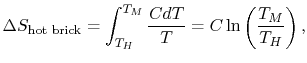where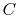is the heat capacity of the brick (J/kg). This quantity is less than zero. For the cold brick,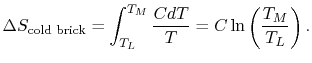The entropy change of the two bricks is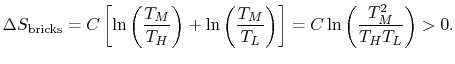The process is not reversible.

5. Difference between the free expansion and the reversible isothermal expansion of an ideal gas

The essential difference between the free expansion in an insulated enclosure and the reversible isothermal expansion of an ideal gas can also be captured clearly in terms of entropy changes. For a state change from initial volume and temperature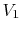,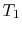to final volume and (the same) temperature,the entropy change is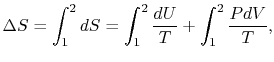or, making use of the equation of state and the fact that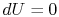for an isothermal process,This is the entropy change that occurs for the free expansion as well as for the isothermal reversible expansion processes �- entropy changes are state changes and the two system final and end states are the same for both processes.

For the free expansion: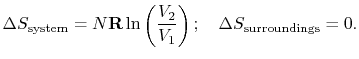There is no change in the entropy of the surroundings because there is no interaction between the system and the surroundings. The total entropy change is therefore,There are several points to note from this result:

1.so the process is not reversible.
2.; the equality betweenandis only for a reversible process.
3. There is a direct connection between the work needed to restore the system to the original state and the entropy change: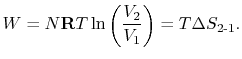The quantityhas a physical meaning as lost work'' in the sense of work which we lost the opportunity to utilize. We will make this connection stronger in Chapter 6.

For the reversible isothermal expansion:

The entropy is a state variable so the entropy change of the system is the same as before. In this case, however, heat is transferred to the system from the surroundings () so thatThe heat transferred from the surroundings, however, is equal to the heat received by the system:.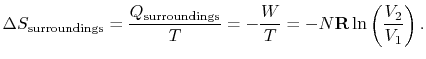The total change in entropy (system plus surroundings) is thereforeThe reversible process has zero total change in entropy.

Muddy Points

On the example of free expansion versus isothermal expansion, how do we know that the pressure and volume ratios are the same? We know for each that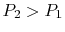and. (MP 5.14)

Where did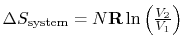come from? (MP 5.15)

UnifiedTP